# Pre-Algebra : Negative Numbers

## Example Questions

### Example Question #1 : Subtract Rational Numbers And Understand The Absolute Value Of Their Difference: Ccss.Math.Content.7.Ns.A.1c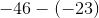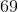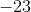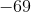Explanation:

When two minus signs meet, the sign becomes positive. Sinceis greater thanand is negative, our answer is negative. We treat as a subtraction problem. Answer is.

### Example Question #3 : Interpret Products Of Rational Numbers And Understand Properites Of Operations: Ccss.Math.Content.7.Ns.A.2a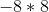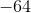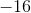Explanation:

When a positive number and a negative number is multiplied, the answer is negative. Just multiply normally. Answer is.

### Example Question #1 : Interpret Products Of Rational Numbers And Understand Properites Of Operations: Ccss.Math.Content.7.Ns.A.2a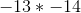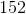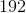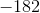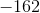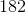Explanation:

When two negative numbers are multiplied, the answer is positive. Multiply normaly. Answer is.

### Example Question #1 : Divide Integers: Ccss.Math.Content.7.Ns.A.2b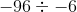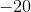Explanation:

When two negative numbers are divided, the answer is positive. Divide normaly. Answer is.

### Example Question #2 : Divide Integers: Ccss.Math.Content.7.Ns.A.2b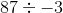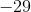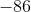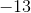Explanation:

When a positive number and a negative number is divided, the answer is negative. Just divide normally. Answer is.

### Example Question #41 : Negative Numbers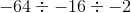Explanation:

There are three negative numbers. If there is an odd number of negative numbers being divided, our answer is negative. Since three is odd, our answer is negative. We divide from left to right. Answer is.

### Example Question #41 : Negative Numbers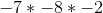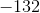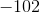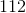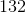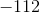Explanation:

There are three negative numbers. If there is an odd number of negative numbers being multiplied, our answer is negative. Sinceis odd, our answer is negative. We multiply from left to right. Answer is.

### Example Question #2 : Add And Subtract Rational Numbers: Ccss.Math.Content.7.Ns.A.1d

Subtract: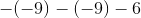Explanation:

Eliminate the double negatives first.

Double negatives will result in a positive sign.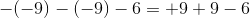Simplify the terms.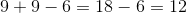### Example Question #41 : Negative Numbers

Solve: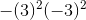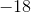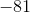Explanation:

In order to evaluate this expression, first square the terms inside the parentheses.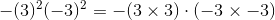Multiply the terms inside the parentheses.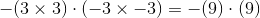Simplify.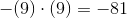### Example Question #1 : Understand Distances Between Numbers On A Number Line: Ccss.Math.Content.7.Ns.A.1b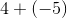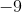Explanation:

When a plus and minus sign meet, we have a minus sign.

Sinceis greater thanand is negative, our answer is negative.

We treat as a normal subtraction.

Answer is.

### All Pre-Algebra Resources